# How and why to hard code values in Excel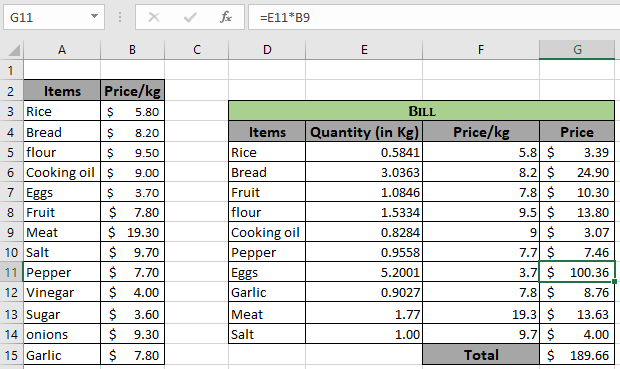In this article, we will learn How and why to hardcode values in Excel.

Scenario:

What is hard coding in Excel. Hard coding is a way of providing values in the formula. For example, if you want to multiply two numbers (11 & 62) in excel, you will either use =11*62 or input 11 in A1 cell and 62 in A2 cell and gets the multiplication in third cell with formula =A1*A2. The formula =11*62 is called the hardcode formula, as you are hard coded 11 and 62 values in the formula. The other way where we used =A1*A2 is called the formula using references. If you want to change the number from 11 to 12 in the formula, then you have to edit the hard code value 11 to 12 wherever required in the formula. But in other case you just have to replace the old value (11) with the new value (12) in the required cell and rest excel handles it. So it's recommended to use references rather than hard coding values in formula in excel

Example :

All of these might be confusing to understand. Let's understand when to use and when not to use Hard code values in formula. Here we have Order quantity in pieces. We need to find the price of the given order quantity using hard code values.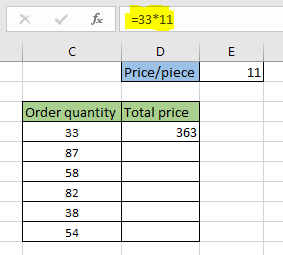The price of the 33 pieces, is 33*11 = 363. Now if i want to multiply each order quantity with the 11 (price/piece). Imagine doing this manually for the data of 10000 orders or more. We cannot copy the formula and paste to other cells as it will return the same result because the values are hard coded as shown below.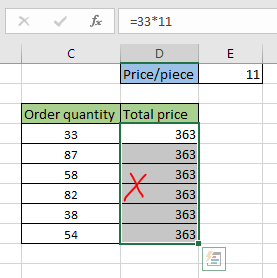Now if use the cell reference for the orders which will be  C4 * 11 = 363. Copying and pasting the formula to other cells will return the right result.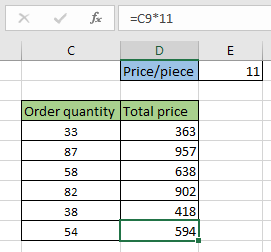In the above image 11 (price/piece) is hard coded value and C4 is the cell reference of the order quantity. Now if i want to change the price/piece to 12. So for this we need to change the formula in first cell and then copy and paste the formula to the other cells which will return the right result. You know this will take some time.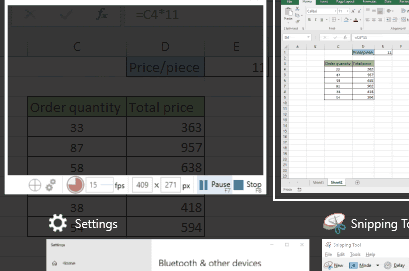But what if we don't hard code price/piece value and use cell reference. For this we will be using the absolute reference for the price/piece value (\$E\$1).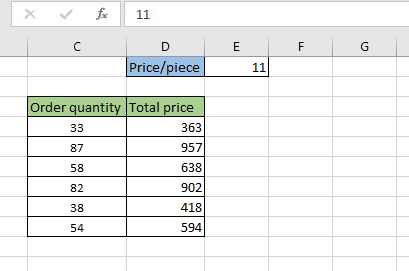In the above gif we can see just changing the value in the E1 cell changes the whole data. This is called using relative and absolute reference instead of hard coding values.

Now let's take an example where we can understand in a much better way why not to use hard code values.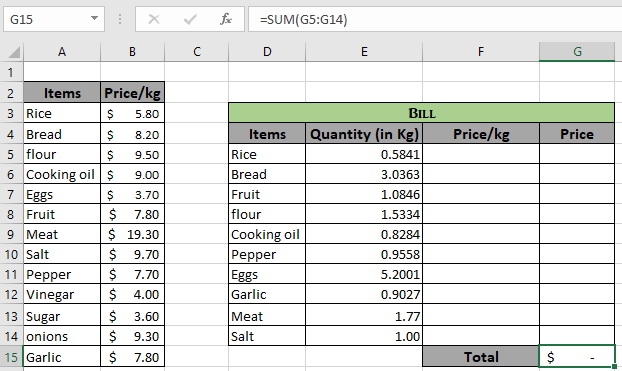We will fetch the result using the VLOOKUP function where we use lookup value, lookup array, index column & match type as arguments. So for this lookup value is given using the relative reference, lookup array using the absolute array reference and the col index & match type as hard code values.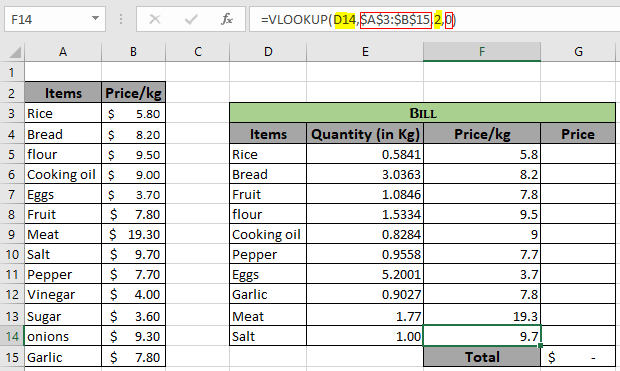As you can see all the arguments in the formula box and how it works in the formula. Now take the product and sum all values or use the SUMPRODUCT function to get the direct sum.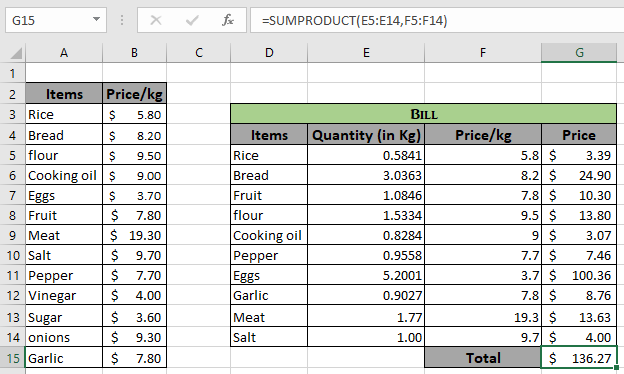SUMPRODUCT function returns the sum and product of each row which will be the total bill.

Here are all the observational notes regarding using the formula.

Notes :

Hard code values are generally used for optional arguments like match_type argument in VLOOKUP function.

Hope this article about How and why to use hard code values in Excel is explanatory. Find more articles on extracting values using references here. If you liked our blogs, share it with your friends on Facebook. And also you can follow us on Twitter and Facebook. We would love to hear from you, do let us know how we can improve, complement or innovate our work and make it better for you. Write us at info@exceltip.com

Related Articles :

All About Excel Named Ranges : excel ranges that are tagged with names are easy to use in excel formulas. Learn all about it here.

The Name Box in Excel : Excel Name Box is nothing but a small display area on top left of excel sheet that shows the name of active cell or ranges in excel. You can rename a cell or array for references.

What is an Absolute Reference in Excel : In many of my articles, you must have read the word absolute reference. If not, you must have noticed the \$ (dollar) sign before cell references (\$A\$1). They are absolute references. When we prefix the \$ sign before.

Expanding References in Excel : The ranges that expand when copied in the below cells or to right cells are called expanding references. Where do we need expanding references? Well, there were many scenarios

Relative and Absolute Reference in Excel : Understanding of Relative and Absolute Reference in Excel is very important to work effectively on Excel. Relative and Absolute referencing of cells and ranges

Dynamic Worksheet Reference : Dynamic worksheet reference suggests that using a value in one sheet from another. The INDIRECT function is a cell reference function that allows us to make the text reference into actual reference.

Popular Articles:

How to use the IF Function in Excel : The IF statement in Excel checks the condition and returns a specific value if the condition is TRUE or returns another specific value if FALSE.

How to use the VLOOKUP Function in Excel : This is one of the most used and popular functions of excel that is used to lookup value from different ranges and sheets.

How to use the COUNTIF Function in Excel : Count values with conditions using this amazing function. You don't need to filter your data to count specific values. Countif function is essential to prepare your dashboard.

How to Use SUMIF Function in Excel : This is another dashboard essential function. This helps you sum up values on specific conditions.

Terms and Conditions of use

The applications/code on this site are distributed as is and without warranties or liability. In no event shall the owner of the copyrights, or the authors of the applications/code be liable for any loss of profit, any problems or any damage resulting from the use or evaluation of the applications/code.